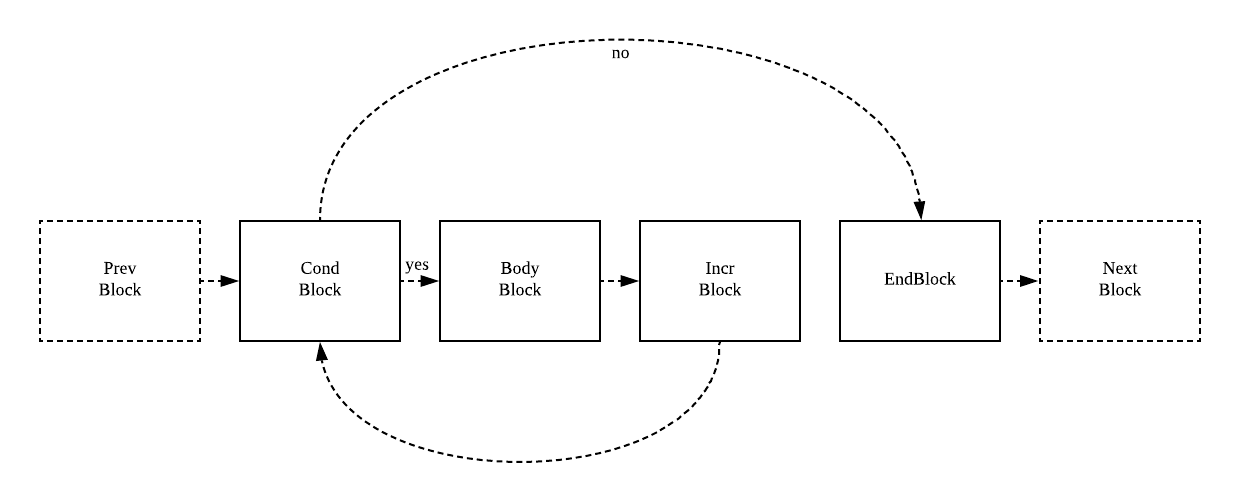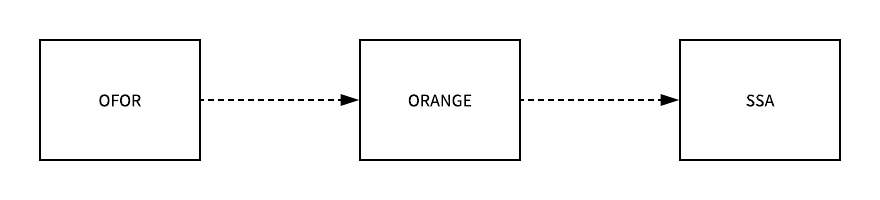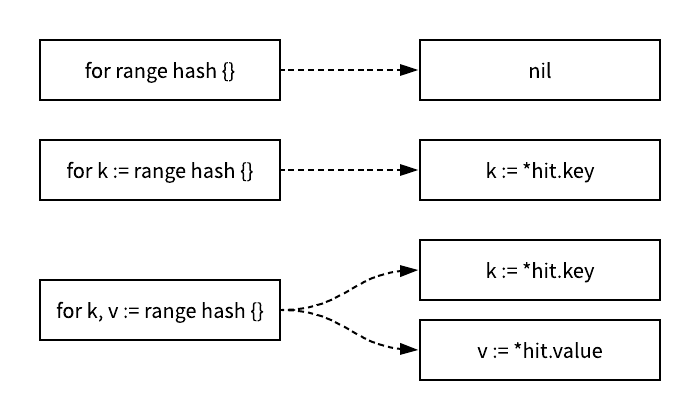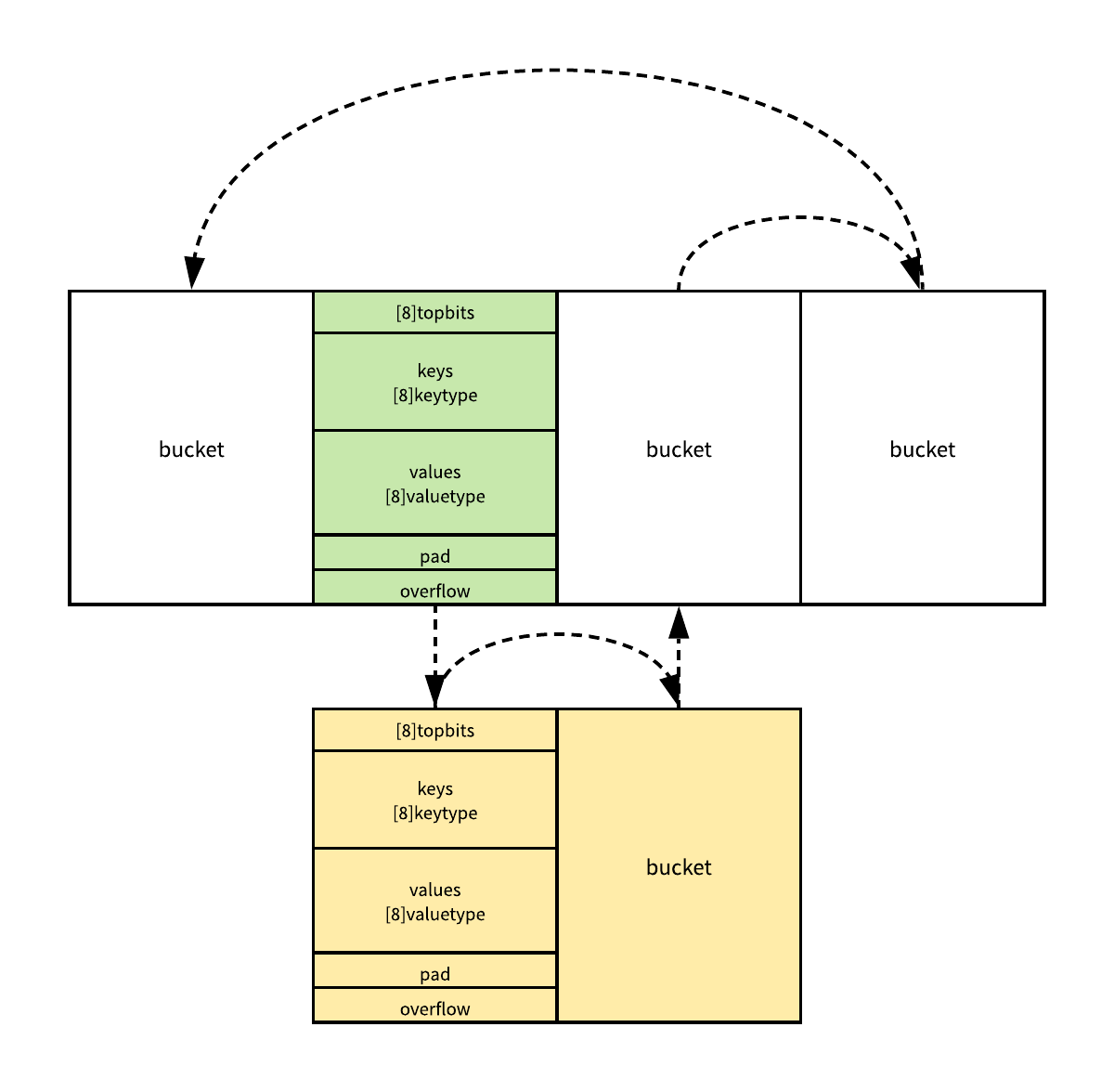# 4.1 for 和 range

## 1. 概述

for 循环能够将代码中的数据和逻辑分离，让同一份代码能够多次复用处理同样的逻辑，我们先来看一下 Go 语言两种不同的 for 循环在汇编代码这一层是如何实现的，首先我们先来看一下经典 for 循环编译之后的底层汇编代码，当我们将一段包含 for 循环的代码编译成汇编语言时会得到如下的结果：

``````\$ cat main.go
package main

func main() {
for i := 0; i < 10; i++ {
println(i)
}
}

\$ go build -gcflags -S  main.go
"".main STEXT size=98 args=0x0 locals=0x18
0x0000 00000 (main.go:3)    TEXT    "".main(SB), \$24-0
# ...
0x001d 00029 (main.go:3)    XORL    AX, AX
0x001f 00031 (main.go:4)    JMP    75
0x0021 00033 (main.go:4)    MOVQ    AX, "".i+8(SP)
0x0026 00038 (main.go:5)    CALL    runtime.printlock(SB)
0x002b 00043 (main.go:5)    MOVQ    "".i+8(SP), AX
0x0030 00048 (main.go:5)    MOVQ    AX, (SP)
0x0034 00052 (main.go:5)    CALL    runtime.printint(SB)
0x0039 00057 (main.go:5)    CALL    runtime.printnl(SB)
0x003e 00062 (main.go:5)    CALL    runtime.printunlock(SB)
0x0043 00067 (main.go:4)    MOVQ    "".i+8(SP), AX
0x0048 00072 (main.go:4)    INCQ    AX
0x004b 00075 (main.go:4)    CMPQ    AX, \$10
0x004f 00079 (main.go:4)    JLT    33
# ...
``````
1. 这段汇编代码其实就是 for 循环被编译之后的结果，存储变量 `i` 的寄存器就是 `AX`，第 0029 行代码对寄存器中的数据进行初始化；
2. 通过 `JMP 75`，跳转到 0075 行，这里会将寄存器中存储的数据与 10 比较并通过 `JLT 33` 命令，在变量的值小于 10 时跳转到 0033 行；
3. 从 0033 行到 0067 行其实都是 for 循环中内部的语句，也就是执行 `println(i)` 打印变量；
4. 每次执行完循环之后都会通过 0072 行中的 `INCQ AX` 指定将变量加一，然后再与 10 进行比较，决定是跳转回循环内部继续执行其中的逻辑，还是顺序执行下面的指令；

``````\$ cat main.go
package main

func main() {
arr := []int{1, 2, 3}
for i, _ := range arr {
println(i)
}
}

\$ GOOS=linux GOARCH=amd64 go tool compile -S main.go
"".main STEXT size=98 args=0x0 locals=0x18
0x0000 00000 (main.go:3)    TEXT    "".main(SB), \$24-0
# ...
0x001d 00029 (main.go:3)    XORL    AX, AX
0x001f 00031 (main.go:5)    JMP    75
0x0021 00033 (main.go:5)    MOVQ    AX, "".i+8(SP)
0x0026 00038 (main.go:6)    CALL    runtime.printlock(SB)
0x002b 00043 (main.go:6)    MOVQ    "".i+8(SP), AX
0x0030 00048 (main.go:6)    MOVQ    AX, (SP)
0x0034 00052 (main.go:6)    CALL    runtime.printint(SB)
0x0039 00057 (main.go:6)    CALL    runtime.printnl(SB)
0x003e 00062 (main.go:6)    CALL    runtime.printunlock(SB)
0x0043 00067 (main.go:5)    MOVQ    "".i+8(SP), AX
0x0048 00072 (main.go:5)    INCQ    AX
0x004b 00075 (main.go:5)    CMPQ    AX, \$3
0x004f 00079 (main.go:5)    JLT    33
# ...
``````

### 1.1. 永不停止的循环

``````func main() {
arr := []int{1, 2, 3}
for _, v := range arr {
arr = append(arr, v)
}
fmt.Println(arr)
}

\$ go run main.go
1 2 3 1 2 3
``````

### 1.2. 神奇的指针

``````func main() {
arr := []int{1, 2, 3}
newArr := []*int{}
for _, v := range arr {
newArr = append(newArr, &v)
}
for _, v := range newArr {
fmt.Println(*v)
}
}

\$ go run main.go
3 3 3
``````

### 1.3. 遍历清空数组

``````func main() {
arr := []int{1, 2, 3}
for i, _ := range arr {
arr[i] = 0
}
}
``````

``````\$ GOOS=linux GOARCH=amd64 go tool compile -S main.go
"".main STEXT size=93 args=0x0 locals=0x30
0x0000 00000 (main.go:3)    TEXT    "".main(SB), \$48-0
# ...
0x001d 00029 (main.go:4)    MOVQ    "".statictmp_0(SB), AX
0x0024 00036 (main.go:4)    MOVQ    AX, ""..autotmp_3+16(SP)
0x0029 00041 (main.go:4)    MOVUPS    "".statictmp_0+8(SB), X0
0x0030 00048 (main.go:4)    MOVUPS    X0, ""..autotmp_3+24(SP)
0x0035 00053 (main.go:5)    PCDATA    \$2, \$1
0x0035 00053 (main.go:5)    LEAQ    ""..autotmp_3+16(SP), AX
0x003a 00058 (main.go:5)    PCDATA    \$2, \$0
0x003a 00058 (main.go:5)    MOVQ    AX, (SP)
0x003e 00062 (main.go:5)    MOVQ    \$24, 8(SP)
0x0047 00071 (main.go:5)    CALL    runtime.memclrNoHeapPointers(SB)
# ...
``````

### 1.4. 随机的遍历顺序

``````func main() {
hash := map[string]int{
"1": 1,
"2": 2,
"3": 3,
}
for k, v := range hash {
println(k, v)
}
}
``````

``````\$ go run main.go
2 2
3 3
1 1

\$ go run main.go
1 1
2 2
3 3
``````

## 2. 经典循环

Go 语言中的经典循环在便一起看来就是一个 `OFOR` 类型的节点，这个节点具有以下的结构，其中包括由于初始化循环的 `Ninit`、循环的中止条件 `Left`、循环体结束时执行的 `Right` 表达式以及循环体 `NBody`

``````for Ninit; Left; Right {
NBody
}
``````

``````func (s *state) stmt(n *Node) {
switch n.Op {
case OFOR, OFORUNTIL:
bCond := s.f.NewBlock(ssa.BlockPlain)
bBody := s.f.NewBlock(ssa.BlockPlain)
bIncr := s.f.NewBlock(ssa.BlockPlain)
bEnd := s.f.NewBlock(ssa.BlockPlain)

b := s.endBlock()
s.startBlock(bCond)
if n.Left != nil {
s.condBranch(n.Left, bBody, bEnd, 1)
} else {
b := s.endBlock()
b.Kind = ssa.BlockPlain
}

s.startBlock(bBody)
s.stmtList(n.Nbody)

s.startBlock(bIncr)
if n.Right != nil {
s.stmt(n.Right)
}
s.startBlock(bEnd)
}
}
``````## 3. 范围循环### 3.1. 数组和切片

``````func walkrange(n *Node) *Node {
switch t.Etype {
case TARRAY, TSLICE:
if arrayClear(n, v1, v2, a) {
return n
}
``````

`arrayClear` 是一个非常有趣的优化，当我们在 Go 语言中遍历去删除所有的元素时，其实会在这个函数中被优化成如下的代码：

``````// original
for i := range a {
a[i] = zero
}

// optimized
if len(a) != 0 {
hp = &a
hn = len(a)*sizeof(elem(a))
memclrNoHeapPointers(hp, hn)
i = len(a) - 1
}
``````

``````        ha := a

hv1 := temp(types.Types[TINT])
hn := temp(types.Types[TINT])

init = append(init, nod(OAS, hv1, nil))
init = append(init, nod(OAS, hn, nod(OLEN, ha, nil)))

n.Left = nod(OLT, hv1, hn)
n.Right = nod(OAS, hv1, nod(OADD, hv1, nodintconst(1)))

if v1 == nil {
break
}
``````

``````ha := a
hv1 := 0
hn := len(ha)
v1 := hv1
for ; hv1 < hn; hv1++ {
// ...
}
``````

``````        if v2 == nil {
body = []*Node{nod(OAS, v1, hv1)}
break
}
``````

``````ha := a
hv1 := 0
hn := len(ha)
v1 := hv1
for ; hv1 < hn; hv1++ {
v1 := hv1
// ...
}
``````

``````        tmp := nod(OINDEX, ha, hv1)
tmp.SetBounded(true)
a := nod(OAS2, nil, nil)
a.List.Set2(v1, v2)
a.Rlist.Set2(hv1, tmp)
body = []*Node{a}
}
n.Ninit.Append(init...)
n.Nbody.Prepend(body...)

return n
}
``````

``````ha := a
hv1 := 0
hn := len(ha)
v1 := hv1
for ; hv1 < hn; hv1++ {
tmp := ha[hv1]
v1, v2 := hv1, tmp
// ...
}
``````

``````func main() {
arr := []int{1, 2, 3}
newArr := []*int{}
for i, _ := range arr {
newArr = append(newArr, &arr[i])
}
for _, v := range newArr {
fmt.Println(*v)
}
}
``````

### 3.2. 哈希

``````ha := a
hit := hiter(n.Type)
th := hit.Type
mapiterinit(typename(t), ha, &hit)
for ; hit.key != nil; mapiternext(&hit) {
key := *hit.key
val := *hit.val
}
````````````func mapiterinit(t *maptype, h *hmap, it *hiter) {
it.t = t
it.h = h
it.B = h.B
it.buckets = h.buckets
if t.bucket.kind&kindNoPointers != 0 {
h.createOverflow()
it.overflow = h.extra.overflow
it.oldoverflow = h.extra.oldoverflow
}

r := uintptr(fastrand())
if h.B > 31-bucketCntBits {
r += uintptr(fastrand()) << 31
}
it.startBucket = r & bucketMask(h.B)
it.offset = uint8(r >> h.B & (bucketCnt - 1))

it.bucket = it.startBucket
mapiternext(it)
}
``````

``````func mapiternext(it *hiter) {
h := it.h
t := it.t
bucket := it.bucket
b := it.bptr
i := it.i
alg := t.key.alg

next:
if b == nil {
if bucket == it.startBucket && it.wrapped {
it.key = nil
it.value = nil
return
}
b = (*bmap)(add(it.buckets, bucket*uintptr(t.bucketsize)))
bucket++
if bucket == bucketShift(it.B) {
bucket = 0
it.wrapped = true
}
i = 0
}
``````

`mapiternext` 函数中第二段代码的主要作用就是从桶中找到下一个遍历的元素，在大多数情况下都会直接操作内存获取目标键值的内存地址，如果当前的哈希表处于扩容期间就会调用 `mapaccessK` 函数获取键值对：

``````    for ; i < bucketCnt; i++ {
offi := (i + it.offset) & (bucketCnt - 1)
k := add(unsafe.Pointer(b), dataOffset+uintptr(offi)*uintptr(t.keysize))
v := add(unsafe.Pointer(b), dataOffset+bucketCnt*uintptr(t.keysize)+uintptr(offi)*uintptr(t.valuesize))
if (b.tophash[offi] != evacuatedX && b.tophash[offi] != evacuatedY) ||
!(t.reflexivekey() || alg.equal(k, k)) {
it.key = k
it.value = v
} else {
rk, rv := mapaccessK(t, h, k)
it.key = rk
it.value = rv
}
it.bucket = bucket
it.i = i + 1
return
}
b = b.overflow(t)
i = 0
goto next
}
``````### 3.3. 字符串

``````ha := s
for hv1 := 0; hv1 < len(ha); {
hv1t := hv1
hv2 := rune(ha[hv1])
if hv2 < utf8.RuneSelf {
hv1++
} else {
hv2, hv1 = decoderune(h1, hv1)
}
v1, v2 = hv1t, hv2
}
``````

### 3.4. 通道

`range` 循环中使用 Channel 其实也是比较常见的做法，一个形如 `for v := range ch {}` 的表达式会最终被转换成如下的格式：

``````ha := a
hv1, hb := <-ha
for ; hb != false; hv1, hb = <-ha {
v1 := hv1
hv1 = nil
// ...
}
``````

## 6. 其他

### 6.2. 微信公众号